Technical Article

# Driving LED Arrays with an Arduino

July 11, 2016 by Cezar Chirila

## All you need to know about LED arrays. If you want to learn about them, drive one using an Arduino, or build them, this is the place to start.

Learn the basics of LED arrays and how to power them using a microcontroller.

If you want to learn how LED displays work and how to power them using microcontrollers, you came to the right place. In this technical article, I will teach you what an LED array is, how the LEDs are connected, and how to drive them efficiently using microcontrollers. I'll use an Arduino as an example.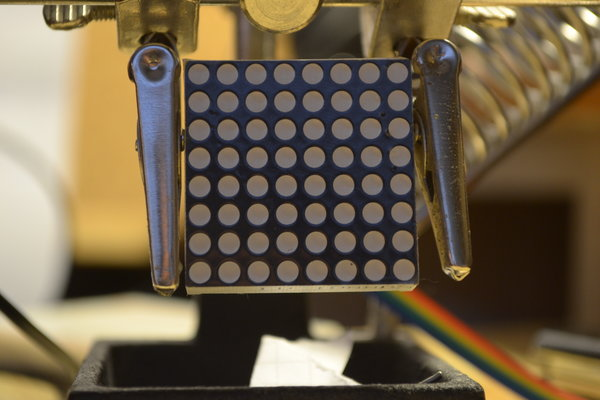### LED Arrays

First of all, what are LED arrays?

Basically, these are displays with each individual pixel being a light-emitting diode. These arrays are widely used in billboards, traffic lights, store signs, and bus destination displays because they are very reliable, consume low amounts of energy, and are easy to manage.

Bigger displays are usually built from multiple modules, each one having its own controller IC. One of the most common LED arrays is the seven-segment display used in applications where you only need to show digits, such as a clock. For example, in the project “Do-It-Yourself Soldering Station with an ATmega8”, I have used a triple seven-segment LED array to display and set the temperature of the soldering iron.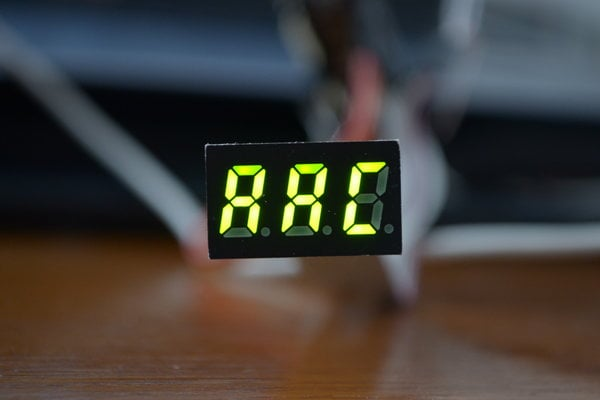### What's Inside Is What Matters Most

To understand how LED arrays work and how to power them, we first need to understand how the LEDs inside are connected.

You might think that the individual LEDs all have a common anode or cathode and that we drive each LED from a microcontroller pin. This is a reasonable assumption for something as simple as a single-digit seven-segment display, but as the number of LEDs in an array increases, this scheme becomes problematic owing to the numerous connections for the individual LEDs. Let’s take for example a standard 8x8 LED matrix: If there were a separate connection for each LED, we would need 64 outputs to control the display. This is definitely not practical.

Below, you have a schematic of how LED arrays are actually built: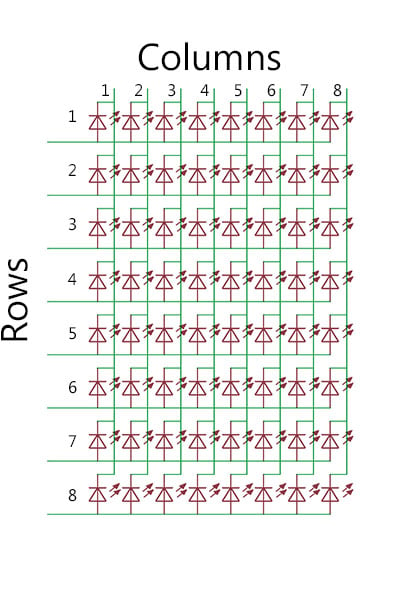The LEDs on the same row have the anode connected together whilst the LEDs on the same column have the cathode in common. Of course, different modules could have the cathode and anode connections switched.

We now have only 16 connections: one for each row and column. When we want to light up a pixel, we apply power to its specific row and column. For example, if we want to turn on LED[1,1], we provide a ground connection for the first column and drive current into the first row. (The format I will use throughout this article is LED[row, column].)

However, a problem appears when we want to light up more LEDs at one time.

### Powering the LED Array

Let’s continue using the same 8x8 LED matrix. I talked about how we can control each individual LED, but a problem arises when we want to light up multiple pixels without lighting up other pixels that share the same anode and cathode connections.

In the gif animation below, you will see exactly what I mean. I want to turn on LED[1,1] and LED[2,2]. Unfortunately, LED[1,2] and LED[2,1] will also light up, forming a square instead of a line.How do we solve this problem?

Simple. We just drive the pixels one at a time and we do it so fast that the human eye cannot tell the difference. To be even more efficient, we can even drive one row at a time without having any problem.

For the matrix above, for example, we scan each row one at a time. This means that we turn on the entire first row, see what LEDs need to be turned on, and ground the columns where we want to turn on the pixels. Then we go to the next row and do the same thing and so on until we reach the last row and start over. This repetition is called "refresh" and how many times it happens in a second is called the "refresh rate", which is measured in Hertz.

This is basically how most displays work. Note that some of them are a bit more complex, such as TVs and monitors, in which the pixels maintain their illumination state and the controllers drive multiple rows at a time.

### Using an Arduino to Power an 8x8 LED Matrix

We have discussed row scanning, in which one entire row is enabled and the LEDs are turned on by grounding the column. You can also use column scanning, in which the entire column is grounded and the LEDs are turned on by applying a voltage to the individual rows.The circuit I have built for this article gives you an example of column scanning.

We will be using an 8x8 LED matrix, the ATmega328 microcontroller, a few passive components, shift registers (74HC595D, TPIC6B596), and the Arduino IDE. You can use the Arduino Uno board or variants if you want.

BOM :

• ATmega328
• LED 8x8 matrix (I used LD-1088BS but you need to check the pinout for your particular matrix)
• 2x22pF capacitors (this is a tentative value; you need to look in your crystal's datasheet and then calculate the proper value for these load capacitors)
• 16MHz quartz crystal
• 10k, 8x200ohm (for the matrix) resistors
• 74HC595D
• TPIC6B596
• 100nF, 100uF 16V capacitors (for filtering the power supply)
• TimerOne library

Just follow the schematic below, or build the LED matrix drive circuit (the right half of the schematic) separately and connect it to the Arduino Uno or variant via 5 wires (VCC, Ground, Data, Clock and Latch). If the drive circuit is connected by wires, it is a good idea to include a 100nF filter capacitor near the power-supply pin of each integrated circuit.

Be careful to note the pinout of your specific LED matrix. Put the 100nF and 100uF capacitors near the microcontroller, between VCC and Ground; check the polarity of the larger capacitor—the case should indicate which lead is negative, and this negative lead must be connected to ground. The resistors R1 to R8 are all 200ohm, but you need to resize them based on the specifications of your LED array following this formula:

$R = \frac{5V-V_{LED}}{I_{LED}}$

where VLED is the typical forward voltage and ILED is the desired forward current.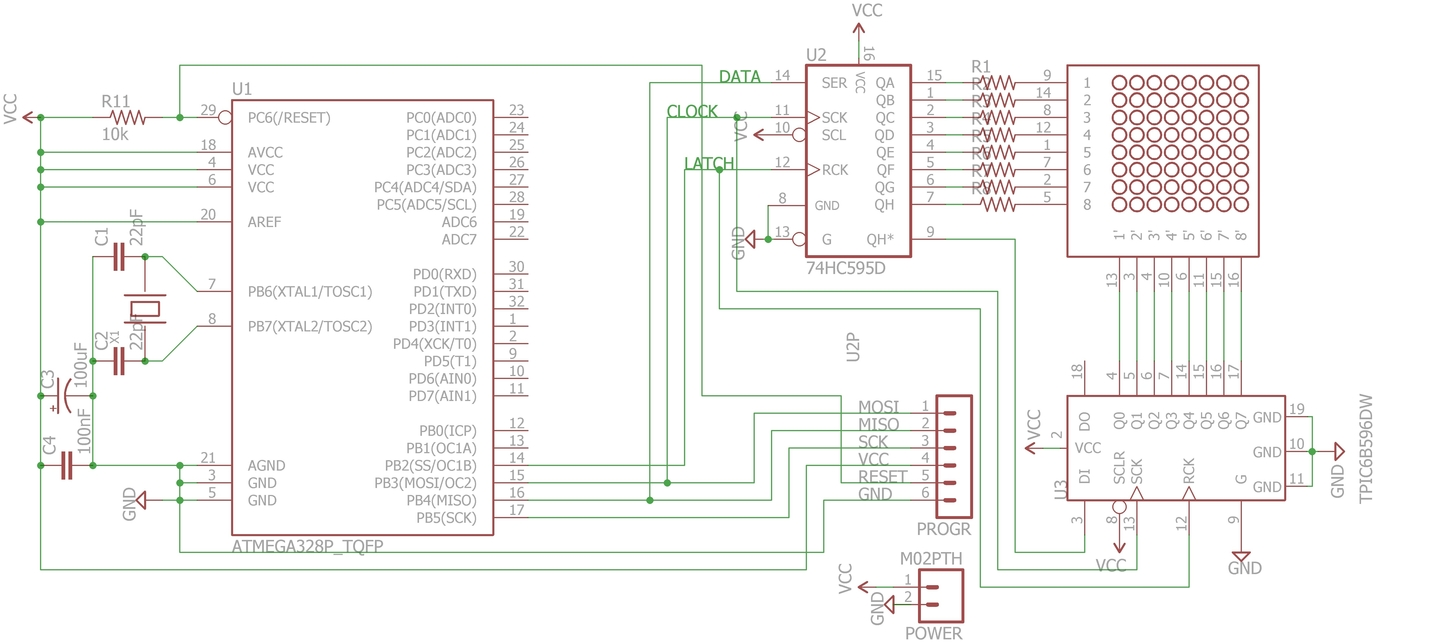Let's now analyse how this circuit works. We have a microcontroller, two shift registers, and an LED array. The two shift registers are daisy chained; this means that they are connected one after the other. After the 74HC595D has received 8 bits, the following ones push the bits that were sent first to the TPIC6B596.

The shift registers receive two bytes of serial data at a time from the microcontroller. These represent first the column and second the rows to be turned on. The serial data is converted into parallel data, with pins QA to QH and Q0 to Q7 being the outputs which are connected to the LED array.

Each bit of data represents the state of each output pin: 1 = VCC in the case of the 74HC595D and 1 = Ground in the case of TPIC6B596 (this is the case for the TPIC6B596 because each output is actually the drain of an N-Channel Mosfet and the bit commands its gate).

Here is an example. Let's say we want to turn LED[2,1] on. We first send the column byte, B00000001, then the row byte, B00000010, which pushes the first byte onto the TPIC6B595, grounding the first column, and the second byte (B00000010) applies VCC to the second row, and so LED[2,1] is on. (These binary numbers correspond to how the bits end up in the shift register; the least significant bit corresponds to QA for the 74HC595D and Q0 for the TPIC6B596.)

PoweringLEDarraysV1.1.zip

The TimerOne library is used to call a function at a specific interval. We use it to precisely control the refresh rate. Here we can see it in action:

void setup() {
...
Timer1.initialize(2000);
Timer1.attachInterrupt(screenUpdate);
}

In this part, we initialize the Timer1 and we specify the time period in microseconds. This time period is the time a column stays enabled until it scans the next one. Each time this period has passed, we call the "screenUpdate" function. To calculate the refresh rate, the total period is eight times greater (because we have 8 columns). Here you can see that I have set a period of 2000µs, which corresponds to a refresh rate of 62.5Hz.

$f = \frac{1}{T} = \frac{1}{2 \cdot 10^{-3}s \cdot 8} = 62.5Hz$

The "screenUpdate()" function is the one that sends the data to the shift registers. It starts with the first column (B00000001) and reads the first byte of the pattern. It then uses the "shiftOut()" function, which is included in the standard Arduino library, to send these two bytes. The "1" in the column byte is shifted and then it reads the second byte of the pattern and so on, until it reaches the last column (B10000000). After this one, the column byte goes back to B00000001 and the process repeats itself.

To edit the display patterns, just edit the "patterns.h" file, and to change the time period for each pattern, change the value of the "interval" variable.

Here is my quick build: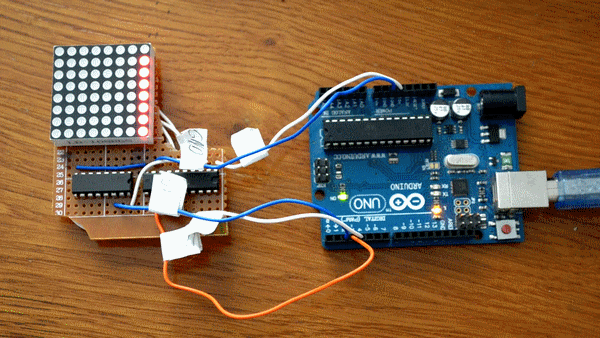### Conclusion

I hope this covers the basics of LED arrays and that you now have now an idea about how they work and how to use them.

Having said that, what are you waiting for? Go buy yourself some LED matrixes or, even better, build yourself one or more (you have the schematic above) and start playing with them. You can easily make an IoT display, LED coffee table, or a Daft Punk helmet.• S
sureshqaz December 30, 2020

I have a query regarding MC9S08QD4
it’s an IC internal RESET and IRQ pin
how it works and how we enable them
And with respect to the GPIO pins of the circuit considering the data from datasheet it has maximum current rating at each pin of 25mA
so while using the IC to take an voltage input an drive two LED for if and else condition (yes or no condition)
the current required for LED alone shall be considered as current consumption or any other considerations.
Can someone help me with it

Like.
• A
Antoine Bourricat February 22, 2021

I have to control an array of several (between 3 and 7) monochromatic colour LEDs. I would like to decide the intensity of each ones once i know which wavelength interests me. It has to be automatic.
Could you propose a mounting with a microcontroller ?

Like.